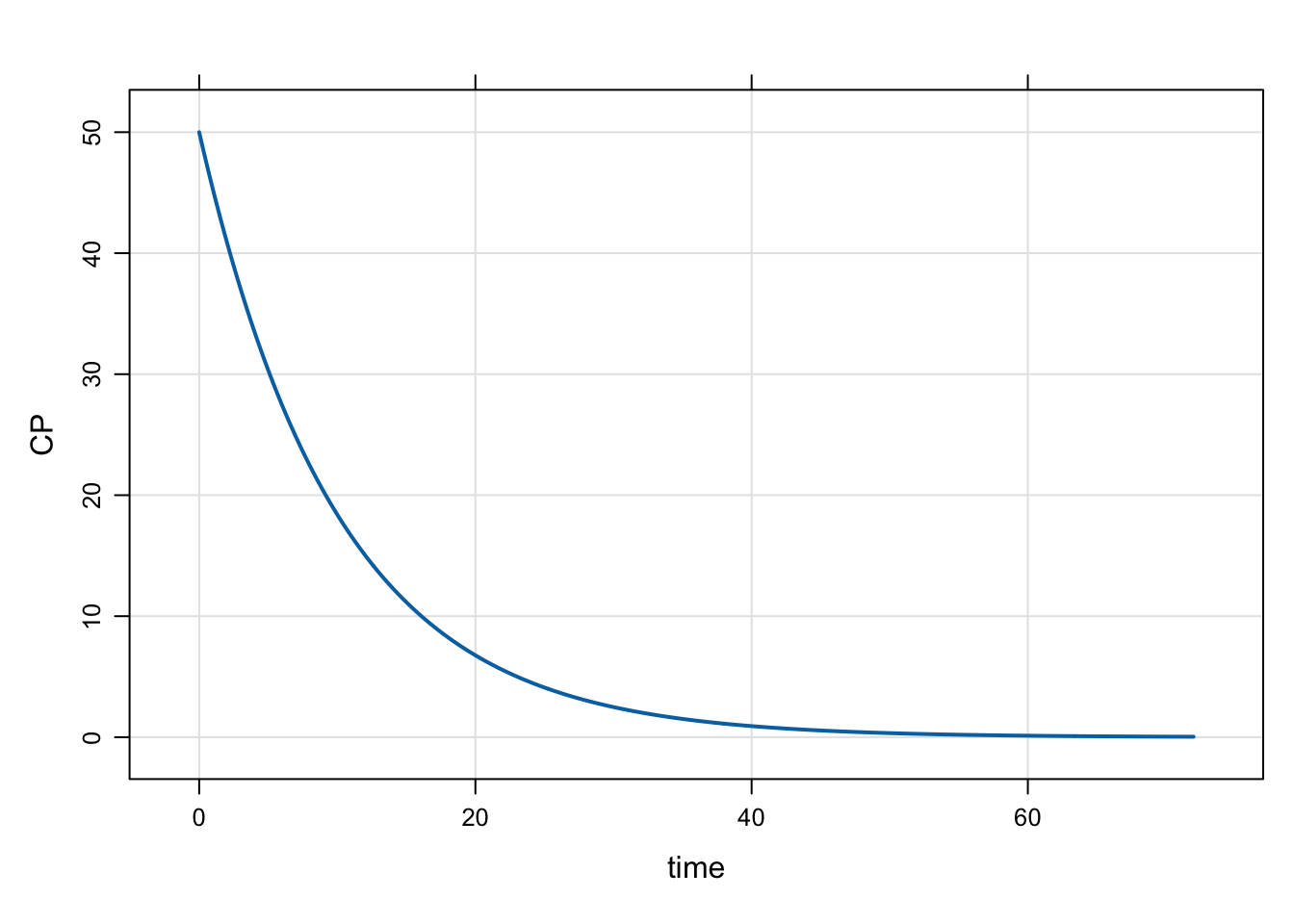Starting with version 0.10.0, mrgsolve can implement dosing records calling for a zero-order infusion at steady state. Find out more in this blog post.

events
infusion
Author

Kyle Baron

Published

January 1, 2020

# 1 Introduction

Starting with version 0.10.0 (released October, 2019), mrgsolve can implement dosing records calling for a zero-order infusion at steady state. To do this, set `rate` to a non-zero value, `amt` to zero, and `ss` to 1.

# 2 Example

For a 10 mg/hr infusion at steady state

``````library(mrgsolve)
library(dplyr)

mod <- house(end = 72, delta = 0.1) %>% update(outvars = "CP")

mod <- param(mod, CL = 2)

dose <- ev(amt = 0, rate = 100, ss = 1, cmt = 2)``````

The dosing event looks like

``dose``
``````Events:
time amt rate cmt evid ss
1    0   0  100   2    1  1``````

With `CL` set to 2, we expect the system to start out at 50

``mrgsim(mod,dose,recsort = 3) %>% plot()``